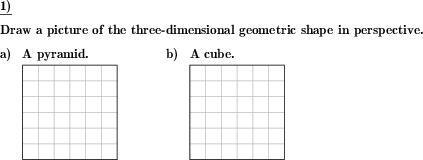Custom math worksheets at your fingertips# Details for problem "Draw 3d geometric shape in perspective"

Quickname: 9522

Elementary School, Primary School, Junior High School, Middle School.

## Summary

Draw an oblique image of the three-dimensional geometric shape given.

## Example## Description

The name of a three-dimensional geometric shape is given. The task is to draw an image of this shape in perspective. This is called an oblique drawing.

These are the shapes that are available:

• a Cuboid or Cube
• a Prism
• a Pyramid
• a Tetrahedron
• a Cylinder
• a Cone

For every shape, it is configurable whether it may appear in the problem statement. The size of the drawing that is shown on the answer sheet can be chosen from two sizes.

An empty raster may be included with the problem statement. The shape can then be drawn on the worksheet and there is need for an extra sheet of squared paper.

Download free printable worksheets for this math problem here. The worksheet contains the problems only, the solution sheet includes the answers. Just click on the respective link.

•Worksheet 1Solution sheet with answers
•Worksheet 2Solution sheet with answers
•Worksheet 3Solution sheet with answers

If you can not see the solution sheets for download, they may be filtered out by an ad blocker that you may have installed. If this is the case, please allow ads for this page and reload the page. The solution sheets will then reappear.

• Do these sample worksheets do not really fit?
• Do you need more math worksheets, with a different level of difficulty?
• Would you like to combine different problems on a worksheet and adjust them to your needs?
• As a teacher, you can put together your own worksheets using the automatically generated math problems provided.
With a free initial credit, you can start creating your own math worksheets in a few minutes.

You can try it for free! Register here, to create custom worksheets now!

## Customization options for this problem

Parameter
Possible values
Number of problems
1, 2, 3, 4, 5, 6
Size of drawing
1, 2, 3
Cube/cuboid
Yes, No
Prism
Yes, No
Tetrahedron
Yes, No
Pyramid
Yes, No
Cylinder
Yes, No
Cone
Yes, No
Empty raster provided
Yes, No

## Similar problems

Remark
Description
Complete a drawing where only the hidden lines are missing from the drawing
The invisible lines are missing in an an oblique sketch of a geometric shape. Add them to the drawing.
Complete the incomplete drawing where some vertices are missing
Lines are missing in an an oblique sketch of a geometric shape. Add hidden and visible lines to the drawing.
The oblique drawing is given, name the shape
An oblique sketch of a geometric shape is shown. Name the shape.
Calculate surface area and volume of a three-dimensional geometric shape
For three-dimensional geometric shapes, state the formula for the volume and/or surface area, or calculate these values.